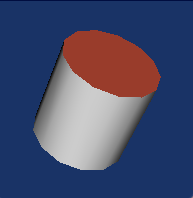# normals of the polydata are messed up after transform

``````   vtkNew<vtkTransform> transform;
transform->PostMultiply();
transform->Scale(1.0, vtkMath::Norm(axisY) / m_Thickness, 1.0);
transform->Concatenate(C);
transform->Update();

vtkNew<vtkTransformPolyDataFilter> transformPolyDataFilter;
transformPolyDataFilter->SetInputData(tubeFilter->GetOutput());
transformPolyDataFilter->SetTransform(transform);
transformPolyDataFilter->Update();

vtkNew<vtkTriangleFilter> triangle;
triangle->SetInputData(transformPolyDataFilter->GetOutput());
triangle->Update();

vtkNew<vtkPolyDataNormals> normalGenerator;
normalGenerator->SetInputData(triangle->GetOutput());
normalGenerator->ComputePointNormalsOn();
normalGenerator->ComputeCellNormalsOn();
normalGenerator->AutoOrientNormalsOn();
normalGenerator->NonManifoldTraversalOff();
normalGenerator->ConsistencyOff();
normalGenerator->Update();
``````

Code is as above. So I create a tube and then apply transform to it (C is the matrix) and then display the normalGenerator->GetOutput()

But here you can see, one of the face is pointing inward. (Blue means normals are pointing outwards and pink means inwards)

Can anyone helps me with this?
Thank you!

It looks like one of the end faces is wound the wrong way. That would cause vtkPolyDataNormals to produce “inward” normals instead of “outward” normals.

I suspect that vtkTransformPolyDataFilter is not the problem. Instead, I think you might have discovered a bug in vtkTubeFilter: it winds one end cap correctly, but winds the other end cap inside-out.

If you remove vtkTransformPolyDataFilter, but keep vtkPolyDataNormals, do you get the same result? Try the following pipeline:

``````vtkTubeFilter -> vtkTriangleFilter -> vtkPolyDataNormals
``````

If I remove vtkTransformPolyDataFilter, everything looks correct. And If I only remove the Concatenation, it also works. I also tried some combinations of rotation matrix. And some work but some don’t.
Also, I tried to use vtkCylinderSource as well. It works the same as tube. They both have issues.

Thank you!

Can you give a sample `C` matrix that doesn’t work?

For example, I just did this:
transform->RotateX(45);
transform->RotateY(60);
transform->RotateZ(80);

And the Tube’s axis is along z axis

I can confirm that the problem exists. In fact, for some rotations both of the end caps are incorrectly oriented.But the problem goes away with this small change:

``````- normalGenerator->AutoOrientNormalsOn();
+ normalGenerator->AutoOrientNormalsOff();
``````

This is very strange, because rotating the polydata should have absolutely no impact on this setting. This indicates either a bug in vtkTransformPolyDataFilter (which I doubt), or a bug in the AutoOrientNormals code in vtkPolyDataNormals (which seems more likely).

I tried that too.
If it is turned off, the whole object is pointing inwards. If I turn it on, there is this one face pointing inwards.
Is there a good solution for me to make them all pointing outwards? I can simply flip it but not sure if it is always the case

Yeah. In my cases, different rotations make different situations.

It seems the only reason that all normals would point inwards is if the `m_Thickness` in your code is negative.

Just checked, it is positive. The bug is still there even I comment out the scale

You’ll have to show how to reproduce the “all pointing inwards” situation, then.

``````lineSource->SetPoint1(0.0, 0.0, 1.0);
lineSource->SetPoint2(0.0, 0.0, -1.0);
lineSource->Update();

vtkNew<vtkTubeFilter> tubeFilter;
tubeFilter->SetInputData(lineSource->GetOutput());
tubeFilter->SetNumberOfSides(30);
tubeFilter->CappingOn();
tubeFilter->Update();
``````

This is the tube creation
Then I just turned off the AutoOrientNormals

This is the C matrix:

-0.99830902570539681 0.057867716719294471 0.0055151207454731122 -4.1893909586657259 0.053899618858214371 0.95701416540486317 -0.28498897926301042 -0.17257266383903502 0.021769710197448333 0.28420980731869339 0.9585149269269575 6.0625220235805708
0 0 0 1

That’s not a rotation matrix, it has a determinant of -1 instead of +1. How was it computed?

In any case, you can probably fix the issue by using `AutoOrientNormalsOff()` and by adding the following to your pipeline (it doesn’t matter where, any place after vtkTriangleFilter will work).

``````// check if the matrix turns the data inside-out
bool flipped = (C->Determinant() < 0.0);

vtkNew<vtkReverseSense> reverse;
reverse->SetInputData(xxx->GetOutput());
reverse->SetReverseNormals(flipped);
reverse->SetReverseCells(flipped);
reverse->Update();
``````

Yeah! It works! I might screw up the coordinates. But thank you!

Hi David, just some follow ups. I used an OBB Tree for an object, and then use (max, mid, min) as the new axes for the new coordinates. And I found out that (max, mid, min) do not make a right-handed coordinates. This is the issue causing the negative Determinant

Ah. When this happens, one option is to use a different corner to define the bounding box, e.g.

``````   if (vtkMath::Determinant3x3(max, mid, min) < 0.0)
{
for (int i = 0; i < 3; ++i)
{
corner[i] += size*min[i];
min[i] = -min[i];
}
}
``````
1 Like

Thank you!